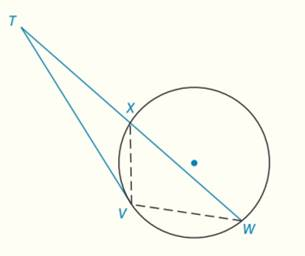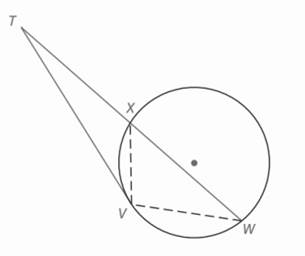Chapter 6.CT, Problem 12CTElementary Geometry For College St...

7th Edition
Alexander + 2 others
ISBN: 9781337614085

Solutions

Chapter
SectionElementary Geometry For College St...

7th Edition
Alexander + 2 others
ISBN: 9781337614085
Textbook Problem

In the figure, T V ¯ is a tangent and Δ T V W ∼   Δ T X V . Find T V if T X = 3 and X W = 5 .To determine

To find:

TV from,.

Explanation

Given:

TV¯ is a tangent and ΔTVWΔTXV.

TX=3 and XW=5.

Theorem used:

“ If a tangent segment and a secant segment are drawn to a circle from an external point, then the square of the length of the tangent equals the product of the length of the secant with the length of its external segment”.

Calculation:

Consider,

From the theorem, “ If a tangent segment and a secant segment are drawn to a circle from an external point, then the square of the length of the tangent equals the product of the length of the secant with the length of its external segment” we can have,

TV2=TXTW(1).

Here,

Still sussing out bartleby?

Check out a sample textbook solution.

See a sample solution

The Solution to Your Study Problems

Bartleby provides explanations to thousands of textbook problems written by our experts, many with advanced degrees!

Get Started

Expand each expression in Exercises 122. (x+1x)2

Finite Mathematics and Applied Calculus (MindTap Course List)

Show that sin1(tanhx)=tan1(sinhx).

Calculus (MindTap Course List)

Solve for if 0360. 3sincos=1

Trigonometry (MindTap Course List)

For a = 2i + 3j − 4k and b = −i + 2j − k, a · b = 0 8 10 12

Study Guide for Stewart's Multivariable Calculus, 8th

True or False: f(t) is used to measure the average rate of change of f with respect to t.

Study Guide for Stewart's Single Variable Calculus: Early Transcendentals, 8th